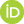# McMule

Yannick Ulrich, Pulak Banerjee, Antonio Coutinho, Tim Engel, Andrea Gurgone, Franziska Hagelstein, Sophie Kollatzsch, Luca Naterop, Marco Rocco, Nicolas Schalch, Vladyslava Sharkovska, Adrian Signer(Monte carlo for Muons and other leptons) is a generic framework for higher-order QED calculations of scattering and decay processes involving leptons. It is written in Fortran 95 with two types of users in mind. First, several processes are implemented, some at NLO, some at NNLO. For these processes, the user can define an arbitrary (infrared safe), fully differential observable and compute cross sections and distributions. McMule’s processes, present and, future, are listed in Table 7 together with the relevant experiments for which the cuts are implemented. Second, the program is set up s.t. additional processes can be implemented by supplying the relevant matrix elements.

 process order experiments comments $$\mu\to\nu\bar\nu e$$ NNLO MEG I&II polarised, massified & exact $$\mu\to\nu\bar\nu e\gamma$$ NLO MEG I polarised $$\mu\to\nu\bar\nu eee$$ NLO Mu3e polarised $$\mu\to\nu\bar\nu e\gamma\gamma$$ LO MEG polarised $$\tau\to\nu\bar\nu e\gamma$$ NLO BaBar cuts in lab frame $$\tau\to\nu\bar\nu l\ell\ell$$ NLO Belle II NLO MUonE NNLO purely electronic corrections mixed (massified) $$\ell p\to\ell p$$ NNLO P2, MUSE, Prad only leptonic corrections $$e^-e^-\to e^-e^-$$ NNLO Prad complete $$e^+e^-\to e^+e^-$$ NNLO no $$n_f$$ $$e^+e^-\to \gamma\gamma$$ NNLO PADME $$e^+e^-\to \mu^+\mu^-$$ NNLO Belle massified

The public version of the code can be found at

To obtain a copy of the code, git is recommended

$git clone --recursive https://gitlab.com/mule-tools/mcmule  Alternatively, we provide a Docker container for easy deployment and legacy results (cf. Section Basics of containerisation). In multi-user environments, udocker can be used instead. In either case, a pre-compiled copy of the code can be obtained by calling $ docker pull yulrich/mcmule  # requires Docker to be installed
\$ udocker pull yulrich/mcmule # requires udocker to be installed


We provide instructions on how is used in Section Getting started.